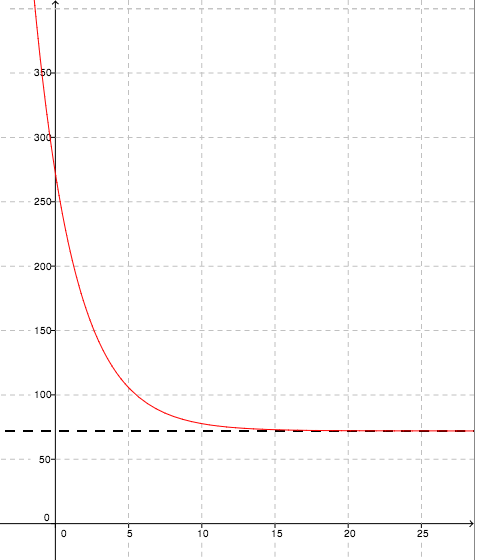### Home > CCA2 > Chapter C > Lesson C.1.2 > ProblemC-34

C-34.

The function $f(x) = 200(0.7)^x + 72$, where $x$ is the time in minutes, could represent the temperature over time of a hot cup of tea placed on the kitchen counter. What does the $72$ represent in the context of this situation? What does the $0.7$ represent?

Separate the function into its component numbers and variables:

$72$: Does this number seem familiar? What is special about $72°$F?

$x$: What purpose does this number serve in the function? What does the $x$-axis represent?

$0.7$: This number gets smaller as $x$ gets bigger. Could it represent a percentage?

$72$ represents room temperature. The cup of hot tea gets continually closer to $72°$ as time progresses. $x$ represents time, probably in minutes, that has passed.
$0.7$ represents the $70\%$ of heat difference that remains after each minute, as $30\%$ of the difference is lost.